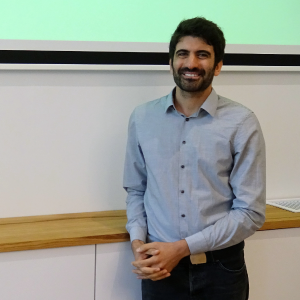# Basic and advanced statistics using XLSTAT, NYC JUNE 16-18 2020

Subscribe to this statistics training course illustrated with XLSTAT Basic+ that will be held in New York City. You will learn the basics of descriptive statistics, multivariate data analysis (PCA, CA, AHC), statistical modeling (ANOVA, regression), statistical tests (parametric and nonparametric) as well as machine learning techniques. All of those essential features will be illustrated using the XLSTAT Basic+ solution.

#### Statistics & Multivariate Analysis with XLSTAT Basic+, 3-day training

This training goes through most commonly used data analysis methods in a wide variety of fields including research, biostatistics, marketing, sensometrics, finance and industry. The course proposes conceptual and intuitive approaches to descriptive statistics, multivariate data analysis, tests, modeling as well as machine learning. Methods are illustrated with many examples and implementations in XLSTAT Basic+, including a thorough interpretation of results. Participants are also given time to practice on real data provided by the trainer. At the end of the training, participants are able to quickly find and implement appropriate statistical methods to answer their own data-related questions, using XLSTAT Basic+.

## Pre-requisites

Basic experience in using Microsoft Excel

## Program

### Introduction

• A couple of definitions: individuals, variables, sample, population

### Describing data:

• Quantitative variables: mean, standard deviation, variance, median, quartiles, Histograms, box plots, scatter plots
• Qualitative variables: frequencies, mode, bar chart, cross tab

### Exploring large data sets:

• Reducing dimensionality: principal component analysis, correspondence analysis
• Segmenting data: agglomerative hierarchical clustering, k-means

### Hypothesis testing

• Defining the null hypothesis, the p-value and error risks
• Parametric tests assumptions
• Parametric tests vs nonparametric tests
• One-tailed tests vs two-tailed tests

### Modeling data

• Linear regression
• One-way ANOVA and multiple comparisons
• Multi-way ANOVA and interaction effects
• ANCOVA

### Machine learning

• Supervised vs unsupervised learning
• Introduction to some supervised machine learning techniques

### Deploying R procedures in Excel

• Overviewing the XLSTAT-R code infrastructure

\$1,300.00

#### 開催地

New York United States

#### Jean-Paul Maalouf